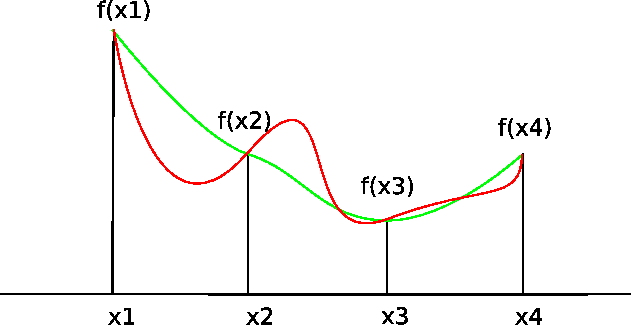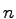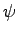## 2. 2. 1 Functions and Discrete Representations

Each function which can be represented in a computer can also be written as linear combination of basis functions of a function space. A weighting vector is given which comprises the single weighting coefficients for the basis functions.

As any other function, such a function has to provide a function value, in general a scalar or a vector value when a point of the simulation domain consisting of coordinates is passed to the function. This holds true for each point within the domain of the function space and not only for a finite selected set of points.

Finite difference schemes often make use of this pointwise formulation. The interpretation of the method (in one dimension) is often described as follows: In the neighborhood of a point it is assumed that the function can be formulated using a truncated Taylor series which is based on the values of this point and the neighboring points, for instance three or five points. After the function is determined by these values, some differential operator is defined in the center point. Figureshows that different interpolating curves are possible and therefore a list of point value pairs is not sufficient in order to characterize the function.The main problem with such a method is that the shape functions are not explicitly given and therefore basic functional evaluations can not be performed in a straight forward manner. In most cases, some interpolation method is found which is used in order to determine the function from the given point-wise values. Mostly, linear interpolation mechanisms are used to recover the function values or the shape of the function so that even differentiation or quadrature can be performed. The determination of distinct function values as a post-processing step is cumbersome and the assumptions of the recovered function are not always compatible with the initial assumptions about the functions used for the discretization.

In analogy, the two- or three-dimensional extension of the Scharfetter Gummel scheme  can be seen for the discretization of the drift-diffusion equations . The Scharfetter Gummel method uses a re-formulation of the functional behavior of the carrier concentrationon an edge in a multi-dimensional space. It can be described as a specialization of the finite difference method which is characterized by a special assumption on the carrier densityrelated to the potential.

If this method is generalized to two or more dimensions using finite volumes - as it is done in several scientific simulation environments  - the values of the function are only defined on the connection lines between two points and it is assumed that the function values do not vary in a direction orthogonal to the connection line. It can be easily shown that such a method does not provide a solution for the complete simulation domain but a function which is defined only on the edges .

Finite element schemes typically define shape functions and use these functions in order to evaluate functionals. From this point of view at each stage of the calculation it is clear which basis functions are used and how they have to be evaluated. A set of coefficients in the computer to identify the function is used within the context of a sum of functions weighted by some stored coefficients. It is therefore clear that also for post-processing steps the same basis functions are chosen and the function is defined consistently with the simulation.

In function spaces which use functions with local support the question of finding basis functions can be reduced to finding an appropriate tesselation of the simulation domain. The elements of the tesselation are used as local support for the respective basis functions. Once an appropriate tesselation is found for a given simulation domain, the function space can be defined in a straight forward manner by defining functions locally on single element of the tesselation.

In order to find an element of the tesselation covering a certain point from this given point, point location'' methods  using jump and walk algorithms  or binary trees like oct-trees and quad-trees [62,63] have to be used. Such a method is required in order to determine which basis functions have to be evaluated in order to determine the function value of a certain point within the simulation domain.

Michael 2008-01-16